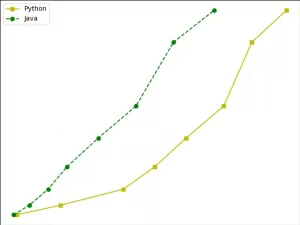# How To Use Matplotlib Axes Classes With Examples

Matplotlib defines an axes class (axis domain class). The objects of this class are called axes objects (i.e. axis domain objects). It specifies a drawing area with value range restrictions. This article will tell you how to use Matplotlib axes classes with some examples.

### 1. Matplotlib Axes Class Introduction.

1. Multiple axes objects can be included in a given figure, but the same axes object can only be used in one figure.
2. The 2D drawing area (axes) contains two axis objects. If it is a 3D drawing area, it contains three.
3. By calling the add_axes() method, we can add axes objects to the canvas. This method is used to generate an axes axis domain object. The position of the object is determined by the parameter rect‘s value.
4. The rect parameter is a position parameter. It accepts a floating-point number list composed of four elements, such as [left, bottom, width, height], which represents the lower-left corner coordinates (x, y), width, and height of the rectangular area added to the canvas.
`ax = figure_object.add_axes([0.1,0.1,0.8,0.8])`
5. The value of each element is a percentage of the canvas width and height. For example, [0.2, 0.2, 0.9, 0.9], it means that painting starts from 20% of the canvas area, and the width and height is 90% of the width and height of the canvas.

#### 1.1 Axes Class legend() Method.

1. The legend() method of the axes class is responsible for drawing the legend on the canvas. It requires three parameters, as shown below.
```ax.legend(handles, labels, loc)

handles : It is a sequence that contains all instances of linetypes.

labels : Is a string sequence that specifies the name of the label.

loc : The parameter that specifies the location of the legend. The parameter value can be expressed as a string or an integer.

the loc parameter's value can be one of the below list.

upper right : upper right or 1.

upper left : upper left or 2.

lower left : lower left or 3.

lower right : lower right or 4.

right : right or 5.

center left : center left or 6.

center right : center right or 7.

lower center: lower center or 8.

upper center : upper center or 9.

center : center or 10.```

#### 1.2 Axes Class plot() Method.

1. This is the basic method of the axes class, which draws the values of one array and the values of another array as lines or markers.
2. The plot() method has optionally formatted string parameters that specify the linetype, marker color, style, and size.
3. Color code table.
```'b' : blue.

'c' : cyan.

'g' : green.

'k' : black.

'm' : magenta.

'r' : red.

'w' : white.

'y' : yellow.
```
4. Marking symbol table.
```'.' : Point mark.

'o' : Circle mark.

'x' : X mark.

'D' : Diamond mark.

'H' : Hexagon mark.

's' : Square mark.

'+' : Plus sign mark```
5. Linetype representation character table.
```'-' : Solid line.

'--' : Dash line.

'-.' : Dotted line.

':' : Dash line.

'H' : Hexagon mark.```

### 2. Matplotlib Axes Example.

1. This example will draw 2 lines with different styles using the axes class’s plot() method.
```import matplotlib.pyplot as plt

def axes_example():

# create a y axis position array.
y = [2, 5, 10, 17, 26,36, 56, 66]

# create 2 x axis position array.
x1 = [2, 16, 36, 46, 56, 68, 77,88]

x2 = [1, 6, 12, 18, 28, 40, 52, 65]

# create a figure object.
figure_object = plt.figure()

# add the axes in the figure object.

# draw a yellow solid curve.
l1 = ax.plot(x1,y,'ys-')

# draw a green circle dash curve.
l2 = ax.plot(x2,y,'go--')

# add a legend on the upper left corner, the legend label is Python & Java.
ax.legend(labels = ('Python', 'Java'), loc = 'upper left')

# show the figure.
plt.show()

if __name__ == '__main__':

axes_example()```
2. When you run it, you will get the below figure.This site uses Akismet to reduce spam. Learn how your comment data is processed.